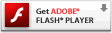# Onebyone Design

## Spinny 3D Trees

I know, I know. Recursive trees are a thing that have been done to death by – well – pretty much everyone.

I just picked up a nice book on Processing the other day though and one of the first examples it gives is a nice recursive tree. In order to better understand the java/processing involved, I thought I’d take the time to convert it to a language I know. Actionscript, that is (in case you were wondering).

After I got it ported and figured out what it was doing and why, I started thinking, that might look kinda cool in 3D. Then I remembered Seb Lee-Delisle had created a ridiculously simple to use 3d drawing api, so I put the two together and came up with the below (roll over to spin the tree around and click to generate a new one).

[kml_flashembed publishmethod=”static” fversion=”10.0.0″ movie=”https://blog.onebyonedesign.com/wp-content/uploads/2010/03/tree.swf” width=”400″ height=”600″ targetclass=”flashmovie”][/kml_flashembed]

If you’d like to have a play with it yourself, the code’s below (and of course you’ll need Seb’s stuff from the link above).

Main

```package {

import com.sebleedelisle.draw3d.Graphics3D;
import flash.display.Sprite;
import flash.events.Event;
import flash.events.MouseEvent;
import flash.text.TextField;
import flash.text.TextFieldAutoSize;
import flash.text.TextFormat;
import geom.Point3D;

/**
* 3D recursive tree based on processing example from
* "Processing: Creative Coding and Computational Art"
* http://www.amazon.com/Processing-Creative-Coding-Computational-Foundation/dp/159059617X/
*
* Using Seb Lee-Delisle Graphics3D lib
* http://sebleedelisle.com/2009/11/simple-flash-3d-drawing-api/
*
*
* @author Devon O.
*/
[SWF(width='400', height='600', backgroundColor='#C0C0C0', frameRate='31')]
public class Main extends Sprite {

public static const DARK_BROWN:uint = 0x5C3317;

private var counter:int = 0;
private var counter2:int = 0;
private var xg:Number = 5;
private var yg:Number = 40;
private var zg:Number = 5;
private var trunkSegments:int = int(Math.random() * 4 + 3);
private var pts:Vector. = new Vector.();
private var branchLimit:int = 325;
private var trunkLength:int = int(Math.random() * 50 + 130);
private var lean2:Vector. = new Vector.(trunkSegments + 1, true);

private var _g3d:Graphics3D;

public function Main():void {
if (stage) init();
}

private function init(e:Event = null):void {

// just some quick text
var tf:TextField = new TextField();
tf.selectable = false;
tf.mouseEnabled = false;
tf.defaultTextFormat = new TextFormat("_sans", 11);
tf.autoSize = TextFieldAutoSize.LEFT;
tf.text = "Click to generate a new tree.";
tf.x = int(stage.stageWidth * .5 + 20);
tf.y = int(stage.stageHeight - tf.height);

_g3d = new Graphics3D(this);

trunk();

}

// set all vars back to original
private function reset():void {
counter = 0;
counter2 = 0;
xg = 5;
yg = 40;
zg = 5;
trunkSegments = int(Math.random() * 4 + 3);
pts = new Vector.();
trunkLength = int(Math.random() * 50 + 130);
lean2 = new Vector.(trunkSegments + 1);
}

private function drawTree(event:MouseEvent):void {
_g3d.clear();
reset();
trunk();
}

private function rotate(event:Event):void {
var ratio:Number = ((stage.mouseX / stage.stageWidth) - .5) * 2;
_g3d.rotateY(ratio * 4);
}

// draws the tree
private function trunk():void {
for (var i:int = 0; i < trunkSegments; i++) {
var lean:Number = randRange(22);
_g3d.moveTo2D(stage.stageWidth / 2 + lean2[i], stage.stageHeight - (trunkLength / trunkSegments) * i, 0);
_g3d.lineTo2D(stage.stageWidth / 2 + lean, stage.stageHeight - (trunkLength / trunkSegments) * (i + 1), 0);
lean2[i + 1] = lean;
}
// set inital branch point from top of trunk
pts = new Point3D(stage.stageWidth * .5 + lean2[trunkSegments], stage.stageHeight - trunkLength, 0);

//create branches
branch(pts);
}

private function branch(pts:Vector.):void {
var stemCount:int = 2;

//  branchLimit controls complexity of tree
if (counter2 < branchLimit){
//set branch thickness
// some conditionals change branches as
// they get further away from the trunk
if(counter2 < 200) {
yg -= Math.random() * .354;
xg -= Math.random() * .625;
} else if (counter2 >= 200) {

// moving into leaf territory now

// at top of tree branches get thinner and more numerous
stemCount = 2 + int(Math.random() * 5);

// leaf color
var leafColor:uint = getColor(Math.random() * 60, 50 + Math.random() * 90, Math.random() * 20);
_g3d.lineStyle(0, leafColor, 230 / 255);

yg -= Math.random() * .75;
xg *= Math.random() * .20;
zg *= Math.random() * .20;
}
for (var j:int = 0; j < stemCount; j++) {

// randomize branch positions
var xx:Number = randRange(30);
var yy:Number = randRange(40);
var zz:Number = randRange(50);

_g3d.moveTo2D(pts[counter2].x, pts[counter2].y, pts[counter2].z);
_g3d.lineTo2D(pts[counter2].x + xg + xx, pts[counter2].y - yg + yy, pts[counter2].z + zg + zz);

// fill up pts array to be passed back recursively to branch function
pts[counter + 1] = new Point3D(pts[counter2].x + xg + xx, pts[counter2].y - yg + yy, pts[counter2].z + zg +zz);

// alternate branches left and right and back and forth
xg *= -1;
zg *= -1;

// keep track of nodes
counter++;
}

// keeps track of branches
counter2++;

//recursive call
branch(pts);
}
}

private function getColor(r:Number, g:Number, b:Number):uint {
return r << 16 | g << 8 | b;
}

private function randRange(val:int):Number {
return Math.random() * val + Math.random() * -val;
}
}
}```

and a simple Point3D class to just store a bit of data

```package geom {
/**
* Basic 3D point
* @author Devon O.
*/
public class Point3D {

private var _x:Number;
private var _y:Number;
private var _z:Number;

public function Point3D(x:Number = 0, y:Number = 0, z:Number = 0) {
_x = x;
_y = y;
_z = z;
}

public function get x():Number { return _x; }

public function set x(value:Number):void {
_x = value;
}

public function get y():Number { return _y; }

public function set y(value:Number):void {
_y = value;
}

public function get z():Number { return _z; }

public function set z(value:Number):void {
_z = value;
}
}
}```

A Saturday morning well spent...

In other news, just discovered this blog's been shortlisted as Best Irish Tech Blog. Some very nice news!